Math Calculators, Lessons and Formulas

It is time to solve your math problem

mathportal.org
• Polynomials
• Graphing Polynomials
• Identifying the graphs of polynomials

# Identifying the graphs of polynomials

ans:
syntax error
C
DEL
ANS
±
(
)
÷
×
7
8
9
4
5
6
+
1
2
3
=
0
.
auto next question
calculator
•  Question 1: 1 pts Which of the following lines could be the graph of a polynomial?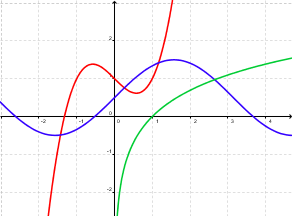green red blue
•  Question 2: 1 pts A graph of the polynomial $p(x)$ is shown in the figure below. Which of the following could be $p(x)$?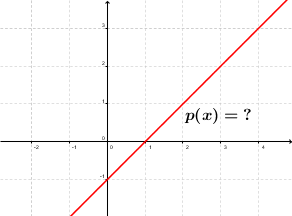$x + 1$ $x -1$ $x^2 +x$ $x^2-x$
•  Question 3: 1 pts The figure on the right shows the graph of a polynomial ? Which of the following could be $p(x)$?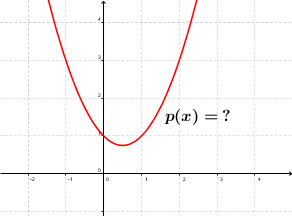$x + 1$ $x^2-x+1$ $x^3+4x$ $x^4 - 3x$
•  Question 4: 2 pts Which of the following lines is NOT the graph of a polynomial?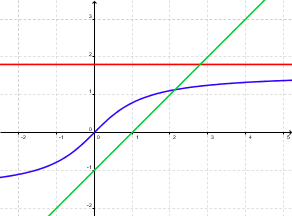green red blue
•  Question 5: 2 pts Which of the following lines could be the graph of a polynomial?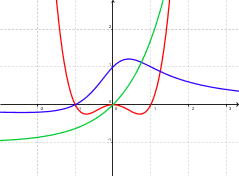green red blue
•  Question 6: 2 pts The degree of the polynomial for which graph is shown at the right is: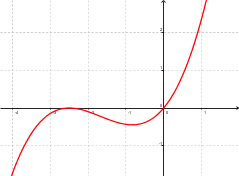odd even
•  Question 7: 2 pts The degree of the polynomial for which graph is shown at the right is: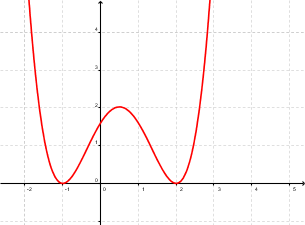odd even
•  Question 8: 3 pts A graph of the polynomial $p(x)$ is shown in the figure below. Which of the following could be $p(x)$?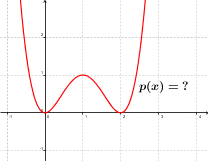$x^2(x-2)^2$ $x^2(x+2)^2$ $x(x+2)$ $x(x-2)$
•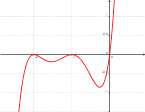Question 9: 3 pts What is the possible degree of the polynomial which graph is shown at the right.
 $3$ $4$ $5$ $6$
•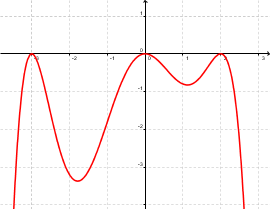Question 10: 3 pts What is the possible degree of the polynomial which graph is shown at the right.
 $3$ $4$ $5$ $6$
•  Question 11: 3 pts Match a given graph to a polynomial to which the graph corresponds.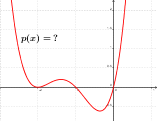$x(x-1)(x-2)^2$ $x(x-1)(x-2)$ $x(x+1)(x+2)$ $x(x+1)(x+2)^2$